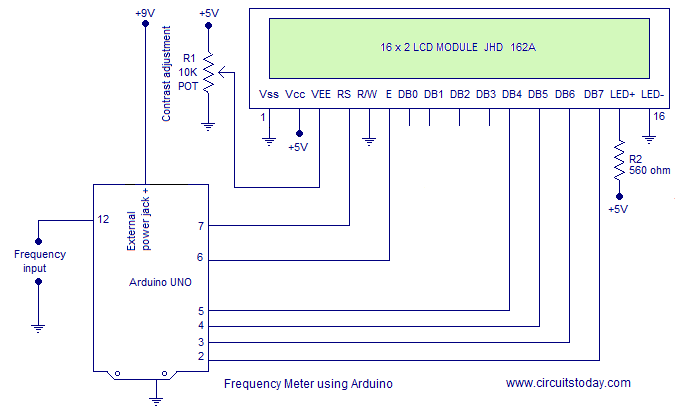## Audio

[Audio][slideshow]

### Frequency counter using Arduino (upto 40KHz).

Many guys here were asking for a frequency counter and at last I got enough time to make one. This frequency counter using arduino is based on the UNO version and can count up to 40KHz. A 16×2 LCD display is used for displaying the frequency count. The circuit has minimum external components and directly counts the frequency. Any way the amplitude of the input frequency must not be greater than 5V. If you want to measure signals over than 5V, additional limiting circuits have to be added and i will show it some other time. Now just do it with 5V signals.

The frequency to be counted is connected to digital pin 12 of the arduino. pulseIn() function is used here for counting the frequency connected to pin 12. pulseIn() function counts the number of pulses (HIGH or LOW) coming to a particular pin of the arduino. The general syntax of this function is pulseIn(pin, value, time) where pin is the name of the pin, value is either HIGH or LOW and time is time for which the function to wait for a pulse. The function returns zero if there is no valid pulse with in the specified time. The pulseIn() function can count pulses with time period ranging from 10 μS to 3 minutes. Circuit diagram of the frequency counter using arduino is given below.Potentimeter R1 is used to adjust the contrast of the LCD screen. Resistor R2 limits the current through the back light LED.

In the program, high time and low time of the input signal is measured using separate pulseIn() functions. Then the high and low times are added together to get the total time period of the signal. Frequency is just 1/time period in seconds. The pulseIn() function returns the time period in microseconds. Total timeperiod in microseconds first divided by 1000. Then 1000 is divided by the result to get the frequency in hertz. The program of the frequency counter using arduino is shown below.

#### Program.

`#include <LiquidCrystal.h>  int input=12;    int high_time;  int low_time;  float time_period;  float frequency;  LiquidCrystal lcd(7, 6, 5, 4, 3, 2);  void setup()  {  pinMode(input,INPUT);  lcd.begin(16, 2);  }  void loop()  {  lcd.clear();  lcd.setCursor(0,0);  lcd.print("Frequency Meter");    high_time=pulseIn(input,HIGH);  low_time=pulseIn(input,LOW);       time_period=high_time+low_time;  time_period=time_period/1000;  frequency=1000/time_period;  lcd.setCursor(0,1);  lcd.print(frequency);  lcd.print(" Hz");  delay(500);  }  `

The circuit can be powered through the 9V external power jack of the arduino. 5V DC required at some parts of the circuit can be tapped from the built in 5V regulator of the arduino itself. This is actually a simple counter circuit using arduino. We can modify this circuit for other applications like tachometer, intrusion counter etc.

[Lights][grids]